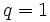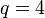# Group cohomology of free abelian groups

This article gives specific information, namely, group cohomology, about a family of groups, namely: free abelian group.
View group cohomology of group families | View other specific information about free abelian group

This article describes the homology and cohomology groups of the free abelian group$\mathbb{Z}^d$ with$d$ generators.

## Classifying space and corresponding chain complex

The free abelian group$\mathbb{Z}^d$ has classifying space equal to the$d$-fold torus, i.e., the space$S^1 \times S^1 \times \dots \times S^1$. A chain complex that can be used to compute the homology for the classifying space and hence also the group is below:

## Homology groups for trivial group action

FACTS TO CHECK AGAINST (homology group for trivial group action):
First homology group: first homology group for trivial group action equals tensor product with abelianization
Second homology group: formula for second homology group for trivial group action in terms of Schur multiplier and abelianization|Hopf's formula for Schur multiplier
General: universal coefficients theorem for group homology|homology group for trivial group action commutes with direct product in second coordinate|Kunneth formula for group homology

### Over the integers$H_q(\mathbb{Z}^d;\mathbb{Z}) = (\mathbb{Z})^{\binom{d}{q}}$

Here,$\binom{d}{q}$ denotes the binomial coefficient: the number of subsets of size$q$ in a set of size$d$. In particular, the homology group is$0$ for$q > d$.

Note that all homology groups themselves are free abelian groups. The homology groups for small values of$d$ and$q$ are given below. Note that missing cells correspond to zero homology groups:$q = 0$$q = 1$$q = 2$$q = 3$$q = 4$$q = 5$$d = 0$$\mathbb{Z}$$d = 1$$\mathbb{Z}$$\mathbb{Z}$$d = 2$$\mathbb{Z}$$\mathbb{Z}^2$$\mathbb{Z}$$d = 3$$\mathbb{Z}$$\mathbb{Z}^3$$\mathbb{Z}^3$$\mathbb{Z}$$d = 4$$\mathbb{Z}$$\mathbb{Z}^4$$\mathbb{Z}^6$$\mathbb{Z}^4$$\mathbb{Z}$

### Over an abelian group

The homology groups with coefficients in an arbitrary abelian group are given below:$H_q(\mathbb{Z}^d;M) = M^{\binom{d}{q}}$